# Overcoming Data Constraints with Transfer Learning

With transfer learning, instead of using an isolated learning approach, a pre-trained large image model is extended with a smaller dataset.

Author: Utsabi Dangol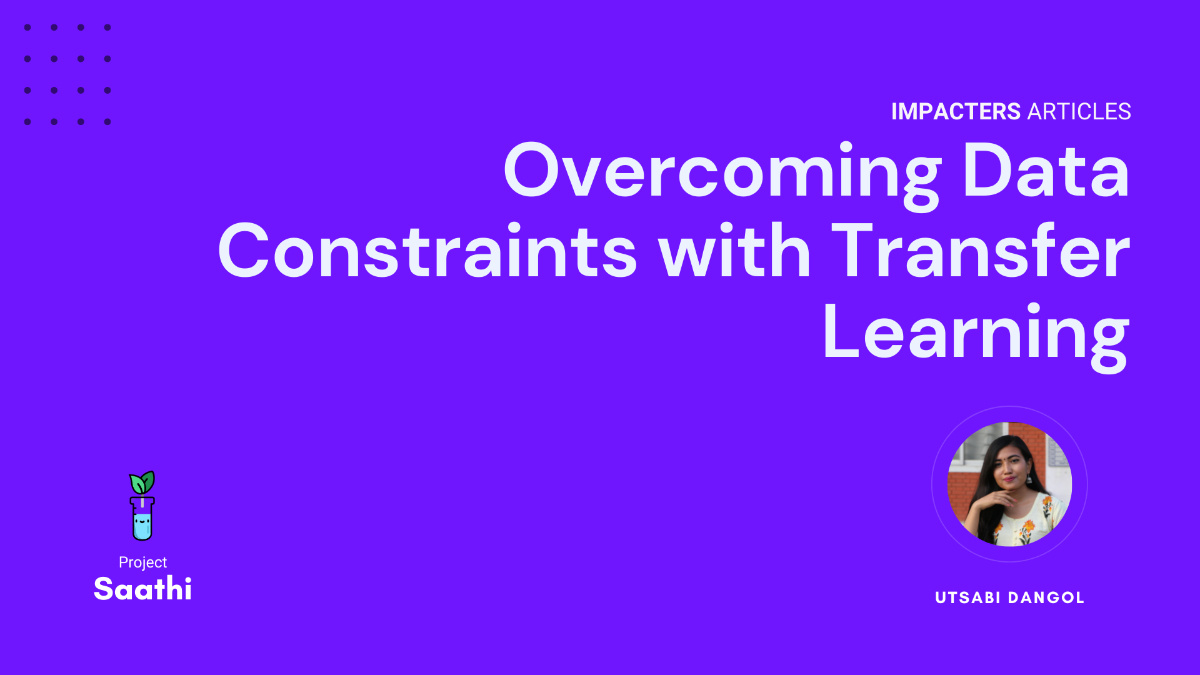Project Saathi is currently working on various machine learning innovations to develop robust and compact edge solutions that encourage Nepali farmers towards data-driven scientific farming methods. Within Saathi, Group iVision specifically deals with predicting crop health based on plant imageries and sensor data. One of the primary goals of iVision is to use machine learning techniques, especially custom neural networks, to predict nutrient deficiency based on images of plants.

One of the biggest known challenges while building a neural network is acquiring enough relevant data for training. Training on small datasets often leads to inaccurate predictions. On the other hand, large datasets add computational complexity to the network making training extremely resource and time-intensive. This is especially problematic if you have to play around with network layers and hyperparameters through multiple training reruns.

Both of these issues can be mitigated to an extent with Transfer Learning. With transfer learning, instead of using an isolated learning approach, a pre-trained large image model is extended with a smaller dataset. This can improve the prediction performance of the models despite the limited dataset and it also saves a lot of time and computational resources as various layers and hyperparameters are explored.

Transfer learning can be applied to different domains including image, text, video, and audio. For images, there are many well-tested models freely available, such as DenseNet, MobileNet, Xception, ResNet, EfficientNet, and VGG. Let us explore some of the basic steps of implementing transfer learning in python and we will later compare some of these pre-trained models available to us.

Implementing Transfer Learning

For the purpose of this article, we will be using the nitrogen deficiency data from . This dataset was collected by an RGB camera (Sony IMX363) from the wheat crop experiment conducted during 2019-20. The folders are arranged in train, test, and validation sets with a split ratio of 70:15:15.

The dataset can be imported into our environment using `ImageDataGenerator` or from the `image_dataset_from_directory`.

``````from tensorflow.keras.preprocessing.image import ImageDataGenerator

BATCH_SIZE = 32
IMG_SHAPE = (224, 224)
train_datagen = ImageDataGenerator(rescale=1 / 255.0)
validate_datagen = ImageDataGenerator(rescale=1 / 255.0)
test_datagen = ImageDataGenerator(rescale=1 / 255.0)
train_data = train_datagen.flow_from_directory(
args["train"],
target_size=IMG_SHAPE,
batch_size=BATCH_SIZE,
class_mode="categorical",
)

validation_data = validate_datagen.flow_from_directory(
args["validate"],
target_size=IMG_SHAPE,
batch_size=BATCH_SIZE,
class_mode="categorical",
)

test_data = test_datagen.flow_from_directory(
args["test"], target_size=IMG_SHAPE, batch_size=BATCH_SIZE, class_mode="categorical"
)``````

``````from tensorflow.keras.preprocessing import image_dataset_from_directory

BATCH_SIZE = 32
IMG_SIZE = (224, 224)
train_dataset = image_dataset_from_directory(
args["train"],
shuffle=True,
batch_size=BATCH_SIZE,
image_size=IMG_SIZE,
label_mode="categorical",
)
validation_dataset = image_dataset_from_directory(
args["val"],
shuffle=True,
batch_size=BATCH_SIZE,
image_size=IMG_SIZE,
label_mode="categorical",
)
test_dataset = image_dataset_from_directory(
args["test"],
shuffle=True,
batch_size=BATCH_SIZE,
image_size=IMG_SIZE,
label_mode="categorical",
)``````

A batch size of 32 is used for this particular scenario. Batch size denotes the number of training data used for one iteration (can also be 64, 128, 256, etc.).

Images are converted to (224, 224) fixed size because in this particular example we are using EfficientNetB0, which was trained on (224, 224) image sizes .

2. Creating models

The pre-trained models can be loaded by specifying the tensorflow hub url directly or by using the `tf.keras.applications` module. We’ll use this to import EfficientNetB0.

``````# Creating model using tensorflow hub efficentnet_url ="https://tfhub.dev/tensorflow/efficientne…"
feature_extractor_layer = hub.KerasLayer(
model_url,
trainable=False,
name="Feature_extraction_layer",
input_shape=IMG_SHAPE(
3,
),
)

# Creating model using tf.keras.application module
import tensorflow as tf

base_model = tf.keras.applications.EfficientNetB0(include_top=False)
base_model.trainable = False``````

The trainable parameter is specified to be false to freeze the base model. Freezing the base model prevents the neural network’s weights from being modified, which eventually decreases the computation and training time. Once the base model is frozen we can create a new model on top of the base model. The model for the given dataset can be created using sequential or functional API.

``````# Using Sequential
model = tf.keras.Sequential(
[
feature_extractor_layer,
layers.Dense(num_classes, activation="softmax", name="output_layer"),
]
)
# Using Functional API
# Create inputs to our model
inputs = tf.keras.layers.Input(shape=(224, 224, 3), name="input_layer")

# Pass the inputs to base model
x = base_model(inputs)
print(f"Shape after passing inputs through base model: {x.shape}")

# Average pool the outputs of base model(aggregate all the most important information,reduce number of computations)
x = tf.keras.layers.GlobalAveragePooling2D(name="global_average_pool_2D")(x)
print(f"Shape after passing inputs through global averagepool: {x.shape}")

# Create the output activation layer
outputs = tf.keras.layers.Dense(
num_classes=2, activation="softmax", name="output_layer"
)(x)

# Combine the inputs with outputs into model
model = tf.keras.Model(inputs, outputs)``````

In general, the functional API is preferred as it is more flexible and the layers can be shared or have multiple inputs and outputs.

3. Compiling the model

Once the model is ready, the model needs to be compiled.

``````model.compile(
loss="categorical_crossentropy",
metrics=["accuracy"],
)``````

4. Fitting the model

The compiled model needs to be trained using the training dataset and validated using validation dataset.

``````history = model.fit(
train_data,
epochs=5,
steps_per_epoch=len(train_data),
validation_data=validation_data,
validation_steps=len(validation_data),
)``````

5. Visualizing the data

Finally, we visualize the results. Visualization is a great way to understand trends and it adds a lot of value to the optimization process. It can give interesting insights on the quality of our model. Visualization also makes it possible to make easy and quick comparisons between different training reruns, so that it is easier to understand the effects of different layers and hyperparameters on the model.

``````def plot_curves(history):
"""Return separate loss curves for training and validation metrics.

Args:
history:Tensorflow History object

Returns:
Plots of training /validation loss and accuracy metrics"""

loss = history.history["loss"]
val_loss = history.history["val_loss"]

acc = history.history["accuracy"]
val_acc = history.history["val_accuracy"]

plt.figure(figsize=(8, 8))
plt.subplot(2, 1, 1)
plt.plot(loss, label="training_loss")
plt.plot(val_loss, label="validation_loss")
plt.title("Training and Validation Loss")
plt.xlabel("epoch")
plt.legend(loc="upper right")
plt.ylabel("Cross Entropy")
plt.show()

plt.subplot(2, 1, 1)
plt.plot(acc, label="Training Accuracy")
plt.plot(val_acc, label="Validation Accuracy")
plt.legend(loc="lower right")
plt.ylabel("Accuracy")
plt.ylim([0, 1])
plt.title("Training and Validation Accuracy")

# Visualizing data
plot_curves(history)``````

6. Result and Analysis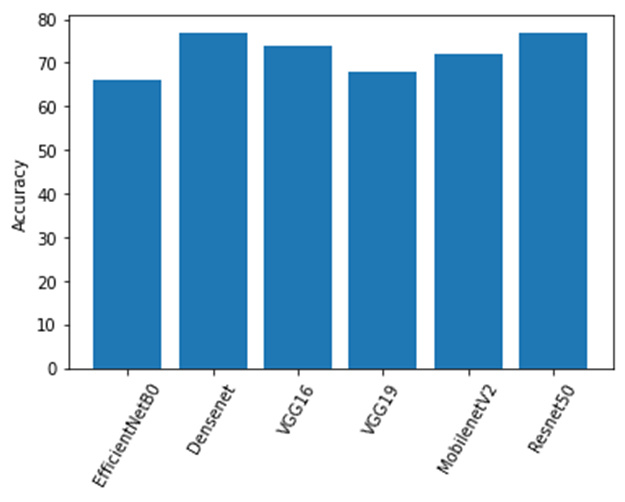By extending the above discussed steps, we trained the dataset on 6 different pre-trained models - EfficientNetB0, Densenet, VGG16, VGG19, MobilenetV2 and Resnet50. The accuracy for the test dataset converged to 66%, 77%, 74%, 68%, 72% and 77% respectively.

These accuracies can be improved by adding more layers, using hyperparameter tuning, and applying fine-tuning.

In general, in our experiment scenario, the EfficientNet models achieved both decent accuracy and better efficiency over existing CNNs, reducing parameter size and FLOPS by an order of magnitude . Therefore, for further detailed analysis and improvement steps EfficientNetB0 was selected.

EfficientNet Plot Graphs

• EfficienctNet‌ ‌for‌ ‌5‌ ‌epochs‌
Without improvement techniques, EfficientNetB0 had an accuracy of 66% for 5 epochs on the test dataset.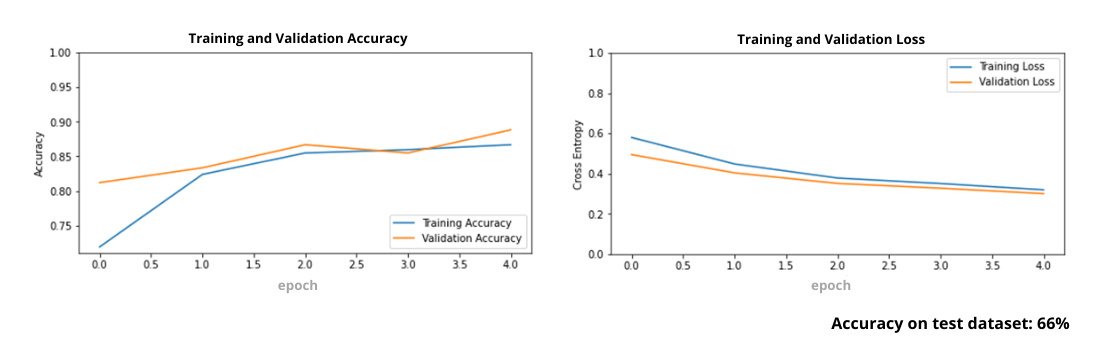• Efficient net with data augmentation (random flip, random rotation, height, width), followed by fine-tuning

Since the dataset is quite small, data augmentation techniques were used to expand the dataset. Data augmentation such as random flip, random rotation, height, and width were applied followed by fine-tuning. However, despite the increase in the size of the dataset, we observed that the validation loss was still increasing. This showed that the data augmentation methods were not working as expected. We therefore decided to remove some of these augmentation techniques.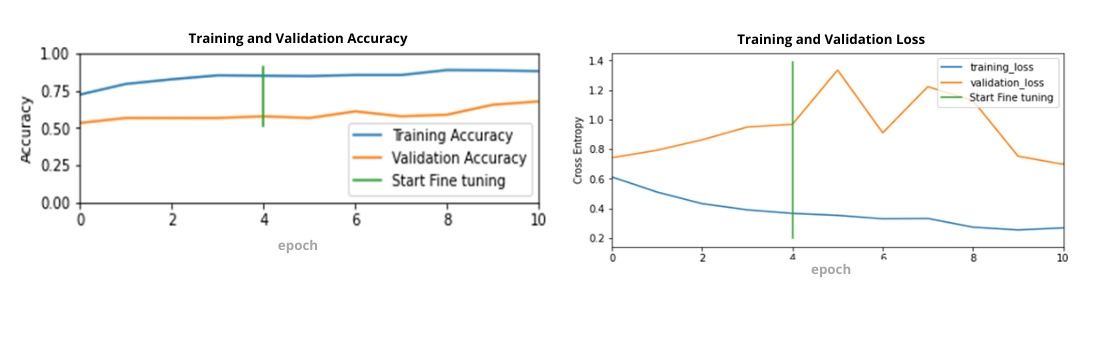• Data augmentation limited to random flip and random rotation

Data augmentation techniques were limited to random flip and random rotation which resulted in the decrease of validation loss.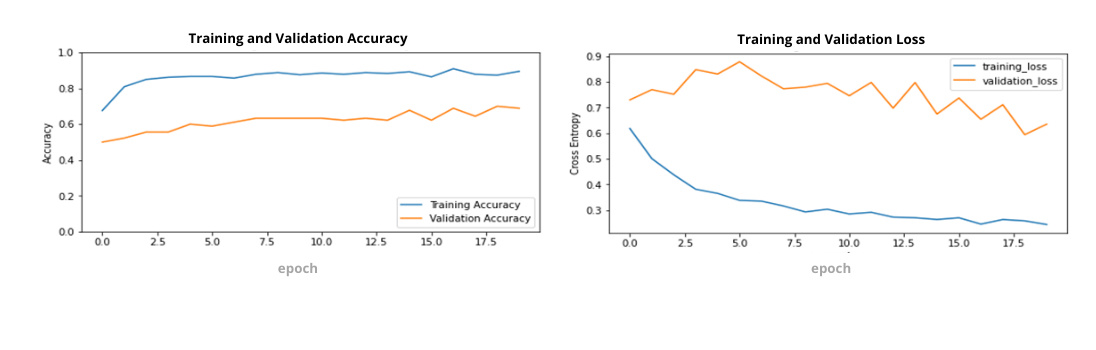• The learning rate changed to 0.001

Learning rate was changed to 0.001, which resulted in accuracy of 65%. Validation loss decreased but the variances still looked very volatile.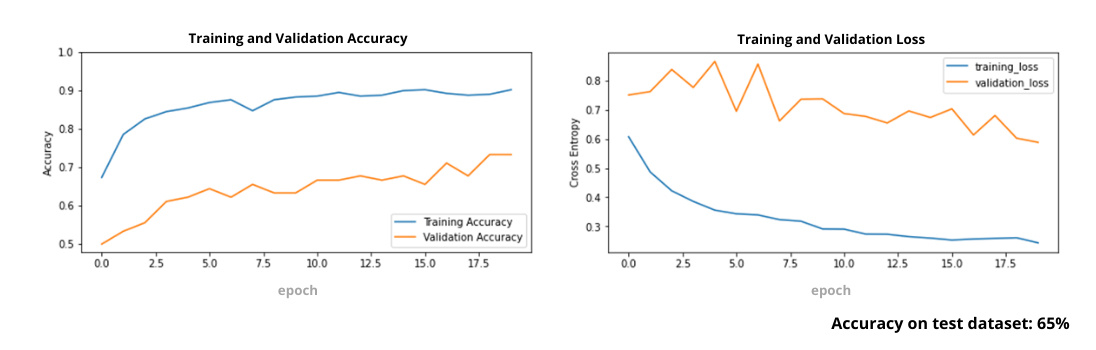• Learning Rate changed to 0.0001

The learning rate was then changed to 0.0001 which reduced the variance volatility. However, the accuracy decreased by 1% to 64%.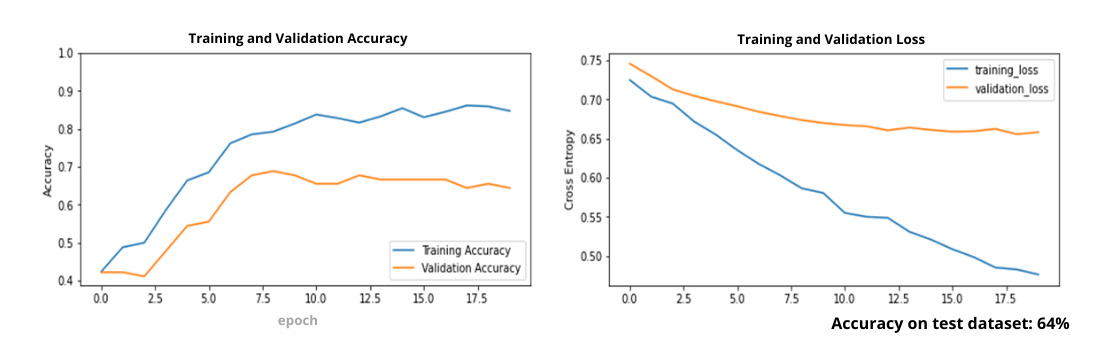• Added 2 Dense layers, enhanced with fine-tuning

The dense layers were added, one with 512 units and another with 128 units. With fine tuning, the accuracy increased from 66% to 83%.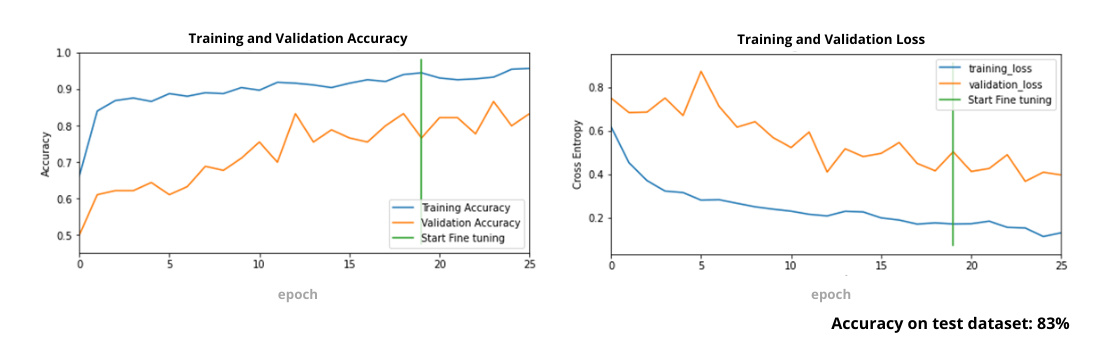Conclusion

In general, improving the accuracy of a neural network requires countless training reruns with different layer structures and parameters. Different methods such as hyperparameter tuning, regularization, and addition of layers can be used to optimize a machine learning model. As such, having the ability to train models faster and with limited resources is an important commodity. Therefore, transfer learning adds a lot of value to a workflow by providing an easier approach to developing machine learning models.

References:

 Arya, Sunny; Singh, Biswabiplab (2020), “Wheat nitrogen deficiency and leaf rust image dataset”, Mendeley Data, V1, DOI: 10.17632/th422bg4yd. 1